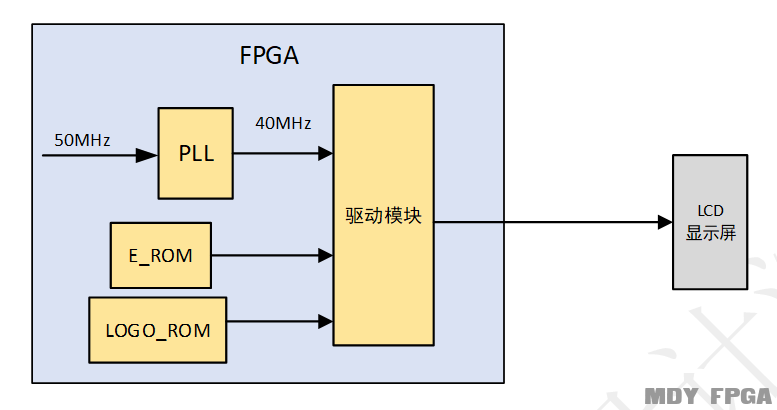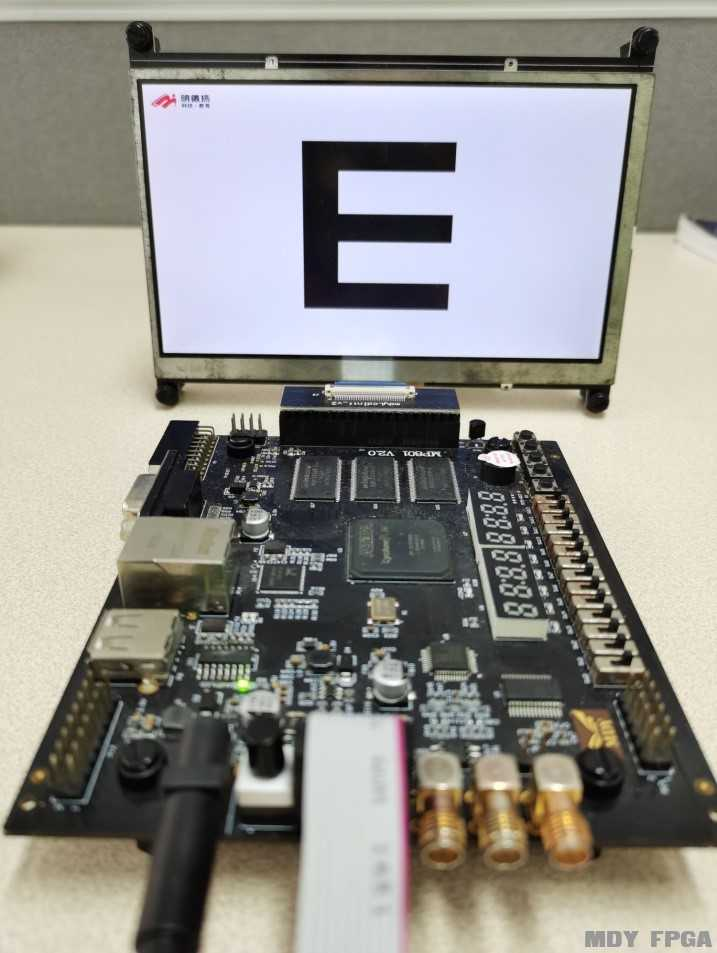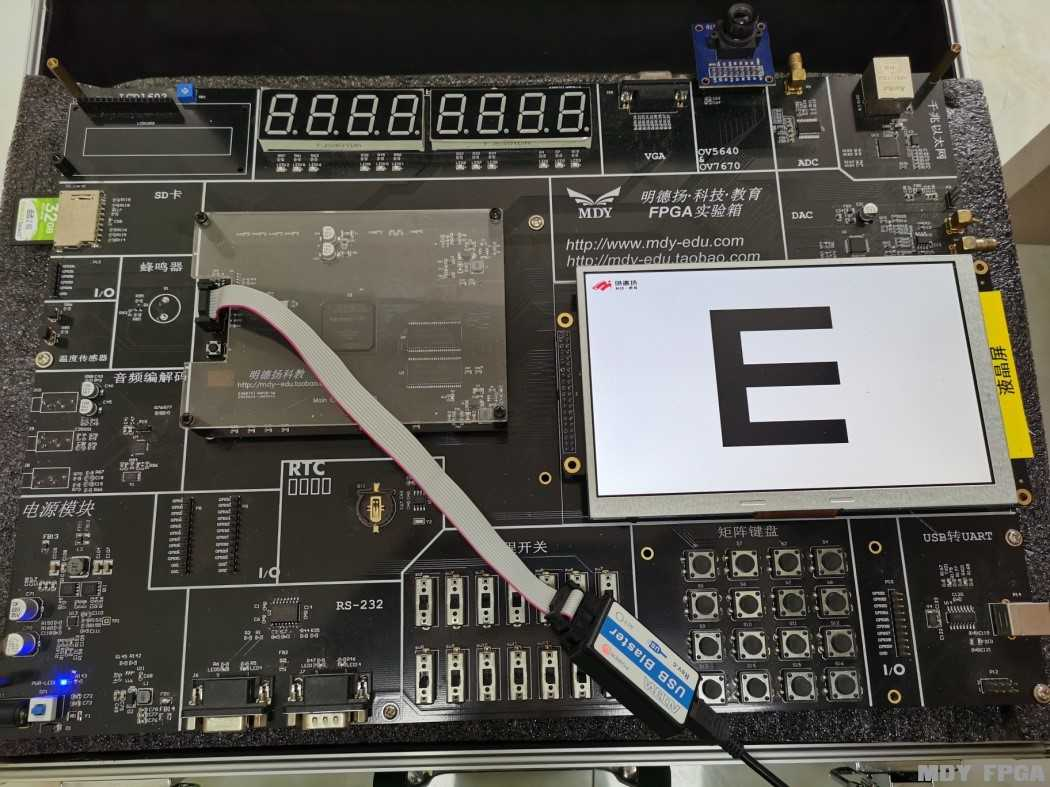【案例】 LCD显示叠加图片

1.1 总体设计

1.1.1 概述

1.1.2 设计目标
7LCD显示屏上实现图片显示。

1.1.3 系统结构框图1.1.4模块功能
PLL模块实现功能
1.       将输入的50MHz时钟分频输出40MHz时钟。

ROM模块实现功能
1.       FPGA_rom存储明德扬LOGO的图像数据；

2.       e_rom存储字母“E”的图像数据。

LCD驱动模块实现功能
1、  产生驱动LCD屏显示的时序
2、  读取ROM里存储的数据并输出显示

1.1.5顶层信号
 信号名 I/O 位宽 定义 clk I 1 系统工作时钟 50M rst_n I 1 系统复位信号，低电平有效 hys O 1 LCD 行时序信号 vys O 1 LCD 场时序信号 lcd_de O 1 LCD 数据输入使能信号 lcd_rgb O 24 LCD RGB信号，RGB格式为使用24位来表示一个像素，RGB分量都用8位表示，取值范围为0-255。 lcd_dclk O 1 LCD 数据采样时钟

1.1.6参考代码
1. module top_mdyLcdPicOverlay(
2.     clk       ,
3.     rst_n     ,
4.     hys       ,
5.     vys       ,
6.     lcd_de    ,
7.     lcd_rgb   ,
8.     lcd_dclk
9.     );

10.     parameter   PICTURE_W = 24  ;

11.     input                   clk         ;
12.     input                   rst_n       ;
13.     output                  hys         ;
14.     output                  vys         ;
15.     output                  lcd_de      ;
16.     output  [PICTURE_W-1:0] lcd_rgb     ;
17.     output                  lcd_dclk    ;
18.

19.     wire                     clk_0      ;
20.
21.     wire                     hys        ;
22.     wire                     vys        ;
23.     wire                     lcd_de     ;
24.     wire   [PICTURE_W-1:0]   lcd_rgb    ;
25.     wire                     lcd_dclk   ;

26. //40MHz
27. pll_40m u_pll_40m(
28.             .areset     (~rst_n ),
29.         .inclk0     (clk    ),
30.             .c0         (clk_0  )
31.     );

32. lcd_driver  u2(
33.    .clk          (clk_0       ),//40MHz
34.    .rst_n        (rst_n       ),
35.
36.    .hys          (hys         ),
37.    .vys          (vys         ),
38.    .lcd_de       (lcd_de      ),
39.    .lcd_rgb      (lcd_rgb     ),
40.    .lcd_dclk     (lcd_dclk    )
41.     );

42. endmodule

1.2 PLL模块设计
1.2.1接口信号

 信号名 I/O 位宽 定义 areset I 1 PLL复位信号，高电平有效 inclk0 I 1 PLL输入时钟 50MHz c0 O 1 PLL输出时钟 40MHz

1.2.2 设计思路

1.3 ROM模块设计
1.3.1接口信号
 信号名 I/O 位宽 定义 address I 16 ROM数据存放地址 clock I 1 ROM工作时钟40MHz q O 8 ROM输出数据

1.3.2设计思路

1.4 LCD驱动模块设计
1.4.1接口信号
 信号名 I/O 位宽 定义 clk I 1 模块工作时钟 40MHz rst_n I 1 系统复位信号，低电平有效 hys O 1 LCD 行时序信号 vys O 1 LCD 场时序信号 lcd_de O 1 LCD 数据输入使能信号 lcd_rgb O 24 LCD RGB信号，RGB格式为使用24位来表示一个像素，RGB分量都用8位表示，取值范围为0-255。 lcd_dclk O 1 LCD 数据采样时钟

1.4.2设计思路

1.4.3参考代码
1. module lcd_driver(
2.     clk          ,//40MHz
3.     rst_n        ,

4.     hys          ,
5.     vys          ,
6.     lcd_de       ,
7.     lcd_rgb      ,
8.     lcd_dclk
9. );

10.    input                    clk             ;
11.    input                    rst_n           ;

12.    output                   hys             ;
13.    output                   vys             ;
14.    output                   lcd_de          ;
15.    output [23:0]            lcd_rgb         ;
16.    output                   lcd_dclk        ;

17.    reg                      hys             ;
18.    reg                      vys             ;
19.    reg                      lcd_de          ;
20.    reg    [23:0]            lcd_rgb         ;
21.    wire                     lcd_dclk        ;

22.    //1056
23.    parameter         THPW      = 20         ;
24.    parameter         THB       = 46         ;
25.    parameter         THD       = 800        ;
26.    parameter         THFP      = 210        ;
27.
28.    //525
29.    parameter         TVPW      = 10         ;
30.    parameter         TVB       = 23         ;
31.    parameter         TVD       = 480        ;
32.    parameter         TVFP      = 22         ;

33.    parameter       HDE_CENTRE  = THD/2      ;//400
34.    parameter       VDE_CENTRE  = TVD/2      ;//240

35.    parameter       LOGO_X0     = (0   + (THB-1))         ;
36.    parameter       LOGO_X1     = (120 + (THB-1))         ;
37.    parameter       LOGO_Y0     = (0   + (TVB-1))         ;
38.    parameter       LOGO_Y1     = (55  + (TVB-1))         ;

39.    parameter         E_X0      = ((HDE_CENTRE-200) + (THB-1))        ;
40.    parameter         E_X1      = ((HDE_CENTRE+200) + (THB-1))        ;
41.    parameter         E_Y0      = ((VDE_CENTRE-150) + (TVB-1))        ;
42.    parameter         E_Y1      = ((VDE_CENTRE+150) + (TVB-1))        ;

43.    reg   [ 10:0]            h_cnt           ;
45.    wire                     end_h_cnt       ;
46.    reg   [ 9:0]             v_cnt           ;
48.    wire                     end_v_cnt       ;

49.    wire                     active_area     ;
50.    reg                      logo_rom_area        ;
52.    wire     [7:0]           logo_rom_data        ;
53.    reg                      e_rom_area        ;
55.    wire     [7:0]           e_rom_data        ;
57.    reg                      e_sel             ;
58.

59. always @(posedge clk or negedge rst_n) begin
60.     if (rst_n==0) begin
61.         h_cnt <= 0;
62.     end
64.         if(end_h_cnt)
65.             h_cnt <= 0;
66.         else
67.             h_cnt <= h_cnt+1 ;
68.    end
69. end
71. assign end_h_cnt = add_h_cnt  && h_cnt == (THB + THD + THFP)-1 ;

72. always @(posedge clk or negedge rst_n) begin
73.     if (rst_n==0) begin
74.         v_cnt <= 0;
75.     end
77.         if(end_v_cnt)
78.             v_cnt <= 0;
79.         else
80.             v_cnt <= v_cnt+1 ;
81.    end
82. end
84. assign end_v_cnt = add_v_cnt  && v_cnt == (TVB + TVD + TVFP)-1 ;

85. /*******************************************************/
86.     //dclk
87.     assign lcd_dclk = clk;

88.     //hsync
89.     always  @(posedge clk or negedge rst_n)begin
90.         if(rst_n==1'b0)begin
91.             hys <= 0;
92.         end
94.             hys <= 1;
95.         end
96.         else if(end_h_cnt)begin
97.             hys <= 0;
98.         end
99.     end

100.     //vsync
101.     always  @(posedge clk or negedge rst_n)begin
102.         if(rst_n==1'b0)begin
103.             vys <= 0;
104.         end
106.             vys <= 1;
107.         end
108.         else if(end_v_cnt)begin
109.             vys <= 0;
110.         end
111.     end
112.

113.     //lcd_de
114.     always  @(posedge clk or negedge rst_n)begin
115.         if(rst_n==1'b0)begin
116.             lcd_de <= 0;
117.         end
118.         else if(active_area)begin
119.             lcd_de <= 1;
120.         end
121.         else begin
122.             lcd_de <= 0;
123.         end
124.     end
125.

126. /********************************************************************/

127. assign active_area = h_cnt>=(THB-1) && h_cnt<(THB+THD-1) && v_cnt>=(TVB-1) && v_cnt<(TVB+TVD-1);

128. always  @(*)begin
129.     logo_rom_area = h_cnt >=LOGO_X0 && h_cnt < LOGO_X1 && v_cnt >= LOGO_Y0 && v_cnt < LOGO_Y1;
130. end

131. always  @(*)begin
132.     e_rom_area = h_cnt >=E_X0 && h_cnt < E_X1 && v_cnt >= E_Y0 && v_cnt < E_Y1;
133. end

134. always  @(posedge clk or negedge rst_n)begin
135.     if(rst_n==1'b0)begin
136.         lcd_rgb <= 0;
137.     end
138.     else if(active_area)begin
139.         if(logo_rom_area)
140.             lcd_rgb <= {logo_rom_data[7:5],5'b11111,logo_rom_data[4:2],5'b11111,logo_rom_data[1:0],6'b111111};
141.         else if(e_rom_area)
142.             lcd_rgb <= {24{e_sel}};
143.         else
144.             lcd_rgb <= {24{1'b1}};
145.     end
146.     else begin
147.         lcd_rgb <=0;
148.     end
149. end

150. always  @(*)begin
151.     logo_rom_addr = (h_cnt-LOGO_X0) + 120*(v_cnt-LOGO_Y0);
152. end

153. always  @(*)begin
154.     e_rom_addr = (h_cnt-E_X0) + 400*(v_cnt-E_Y0);
155. end

156. always  @(posedge clk or negedge rst_n)begin
157.     if(rst_n==1'b0)begin
159.     end
160.     else begin
162.     end
163. end

164. always  @(*)begin
166. end

167. fpga_rom u_fpga_rom(
169.                    .clock   (clk     ),
170.                    .q       (logo_rom_data));

171. e_rom u_e_rom(
173.     .clock     (clk  ),
174.     .q         (e_rom_data  ));

175.     endmodule

1.5 效果和总结

mp801开发板ms980试验箱拓展阅读